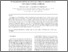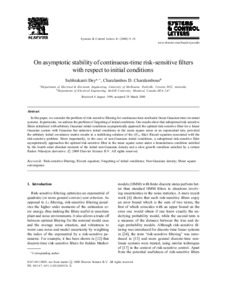# On asymptotic stability of continuous-time risk-sensitive filters with respect to initial conditions

Dey, Subhrakanti and Charalambous, Charalambos D. (2000) On asymptotic stability of continuous-time risk-sensitive filters with respect to initial conditions. Systems and Control Letters, 41 (1). pp. 9-18. ISSN 0167-6911Previewmore...Add this article to your Mendeley library

## Abstract

In this paper, we consider the problem of risk-sensitive filtering for continuous-time stochastic linear Gaussian time-invariant systems. In particular, we address the problem of forgetting of initial conditions. Our results show that suboptimal risk-sensitive filters initialized with arbitrary Gaussian initial conditions asymptotically approach the optimal risk-sensitive filter for a linear Gaussian system with Gaussian but unknown initial conditions in the mean square sense at an exponential rate, provided the arbitrary initial covariance matrix results in a stabilizing solution of the (H∞-like) Riccati equation associated with the risk-sensitive problem. More importantly, in the case of non-Gaussian initial conditions, a suboptimal risk-sensitive filter asymptotically approaches the optimal risk-sensitive filter in the mean square sense under a boundedness condition satisfied by the fourth order absolute moment of the initial non-Gaussian density and a slow growth condition satisfied by a certain Radon–Nikodym derivative.

Item Type: Article Risk-sensitive filtering; Riccati equation; Forgetting of initial conditions; Non-Gaussian density; Mean square convergence; Faculty of Science and Engineering > Electronic EngineeringFaculty of Science and Engineering > Research Institutes > Hamilton Institute 14409 https://doi.org/10.1016/S0167-6911(00)00030-X Subhrakanti Dey 10 May 2021 15:42 Systems and Control Letters Elsevier YesItem control page